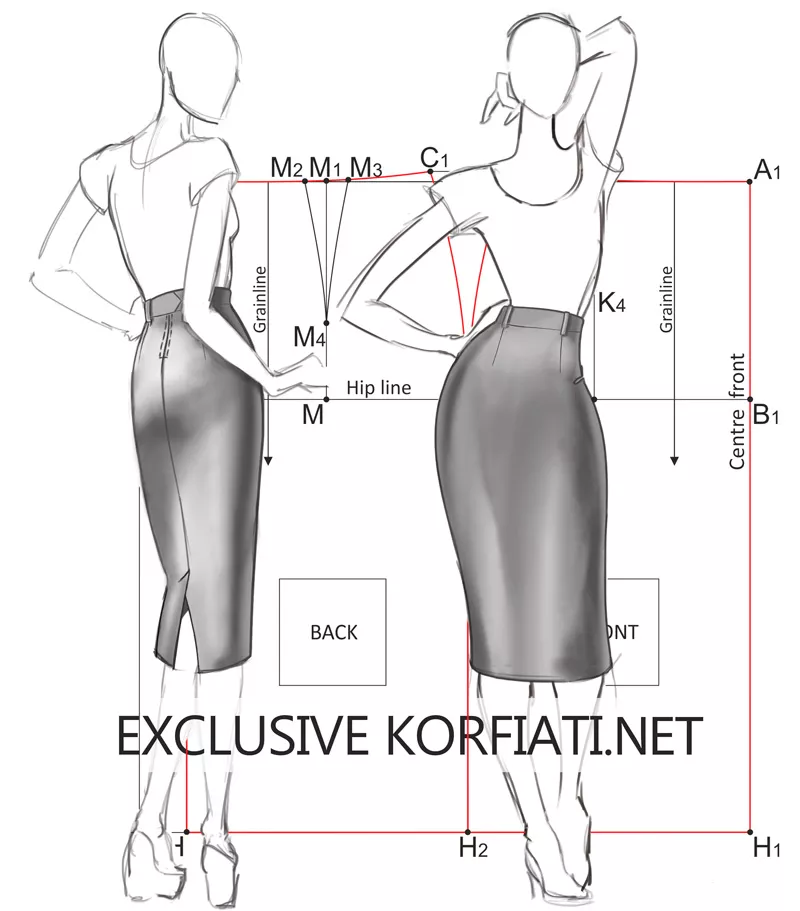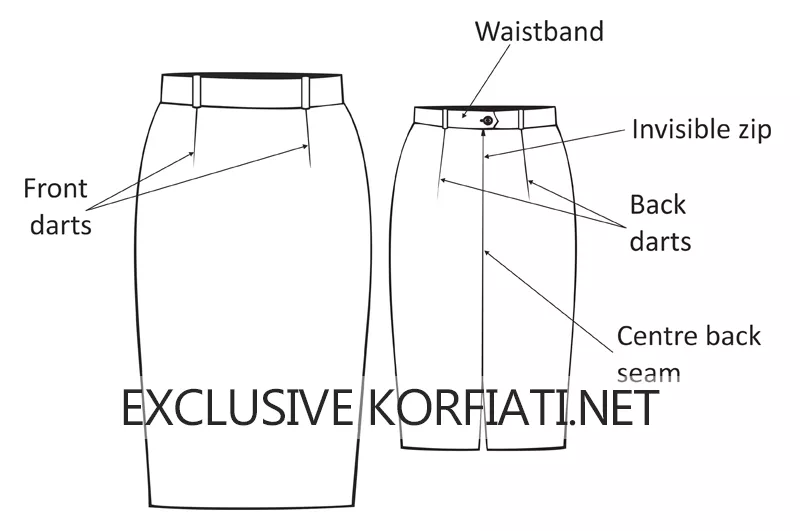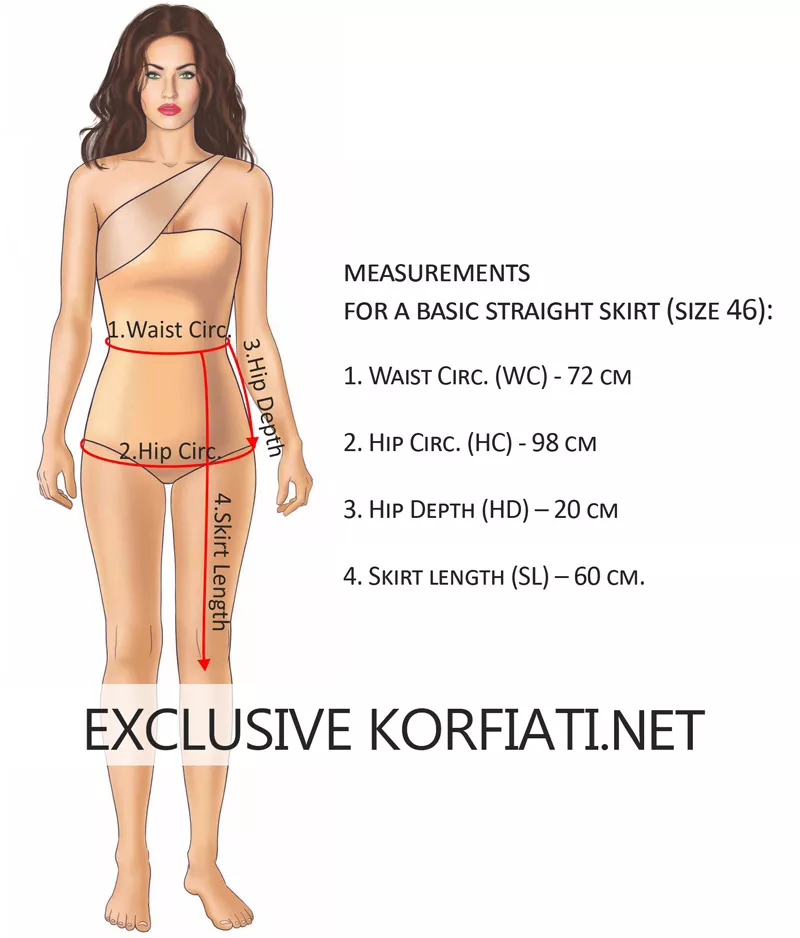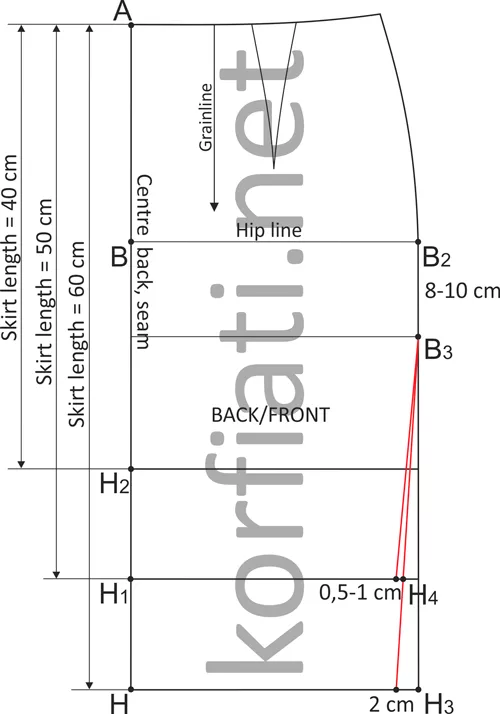• 2

# Basic straight skirt

## Basic straight skirt – pattern with dartsSewing any skirt begins with the construction of the basic pattern. We recommend to draw a basic skirt on graph paper. In order to obtain a perfect result, measurements need to be taken оn a person, dressed with underwear. Basic straight skirt pattern – in this tutorial.

Contents:

Sketch of the skirt is on the pic. 1. Straight skirt has details: front and back darts, invisible zip in the center back seam, waistband.To contents ▴

## Basic straight skirt

Measurements needed for a basic straight skirt (size 46):

1. Waist circ. (WC)- 72 cm
2. Hip circ. (HC)- 98 cm
3. Hip depth (HD) – 20 cm
4. Skirt length (SL) – 60 cm.Pic. 2. Measurements for basic straight skirt

To contents ▴

#### How to draw a skirt pattern

Draw a rectangle АА1НН1:

1. АА1 = HH1 = ½ Нip circ + 1 cm = 98/2 + 1 cm = 49 cm + 1 cm = 50 cm.
2. AH = A1H1 = 60 cm (Skirt Length).
3. AA2 = A2A1 = ½ AA1 = 50/2 = 25 cm. From the point A2 square down to join HH1 – point H2.
4. AB = 20 cm (Hip length measurement). From point B square wright to join A1H1 – point B2.Pic. 3. Basic straight skirt

Darts calculation: ½ Hip circ. – ½ Waist circ. = 49 cm – 36 cm = 13 cm.

• Side seam dart calculation: (½ Hip circ. – ½ Waist circ.) /2 = (49 cm – 36 cm)/2 = 13 cm /2 = 6,5 cm.
• Back dart calculation: (13 cm – 6,5 cm) * 0,6 = 3,9 cm ≈ 4 cm.
• Front dart calculation: (13 cm – 6,5 cm) * 0,4 = 2,6 cm ≈ 2,5 cm.

A2C = 1 cm (square up). Side seam: C1C2 = 6,5 cm (from point C move 3,25 cm each side). Join points C1 and C2 to point B2, using a curve.

To contents ▴

#### How to draw a Back dart

Point M = ½ BB2. Square up to join AA2 – point M1. From point M1 move 2 cm each side: M1M2 = M1M3 = 2 cm. Back dart length: M1M4 = 13 cm.
Join points M2 and M3 to point M4, using a curve. Join point M3-C1 using a curve.

#### How to draw a Front dart

From point C2 move right 8 cm: C2K = 8 cm (not a fix measurement, may be increase). Square down KK1. From point K move 1,25 cm each side: KK2 = KK3 = 1,25 cm. Front dart length: KK4 = 10 cm. K4K5 – move left 0,5 cm. Join points K2 – K3 – K5, using a curve. Waist line: join point K2-C2 using a curve.

#### Back skirt pattern with 4 darts

A back with 4 darts is used in the case of a small waist (if Hip circ. minus Waist circ > 14 cm). Back pattern is on pic. 2. Front cut out unchanged (see pic. 3).Pic. 4. Back skirt pattern with 4 darts

To contents ▴

#### Pencil-skirt modeling

The pencil-skirt is a very popular and has a fit silhouette. The principle of a basic pattern transforming is shown in pic. 5.Pic. 5. Pencil-skirt modeling

There are more patterns and ideas for creativity on the site of the Anastacia Korfiati Sewing School! Subscribe free!